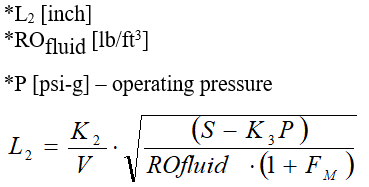ASME PTC 1974 - Intergraph Smart Instrumentation - 13.1 - Reference - Hexagon PPM

Integraph Smart Instrumentation Sizing Equations

Language
English (United States)
Product
Intergraph Smart Instrumentation
Search by Category
Reference
Smart Instrumentation Version
13.1
1. Define initial value of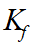constant.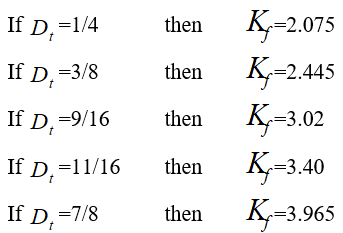2. Calculate Ratio of Frequency at Fluid Temperature to Frequency at 70 °F: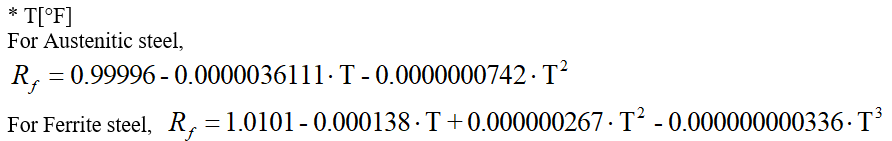3. Calculate Maximum Thermowell Length Based on Vibration Limitation: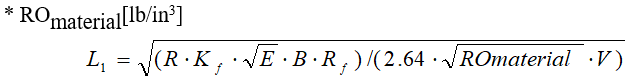4. DefineConstant: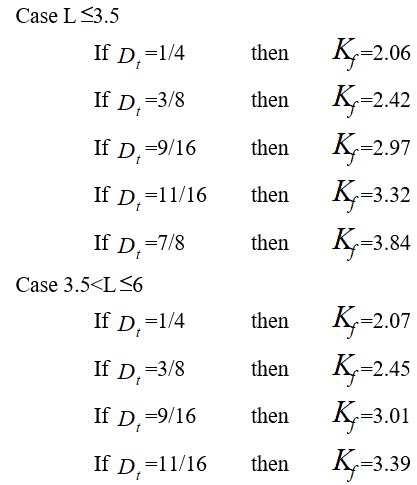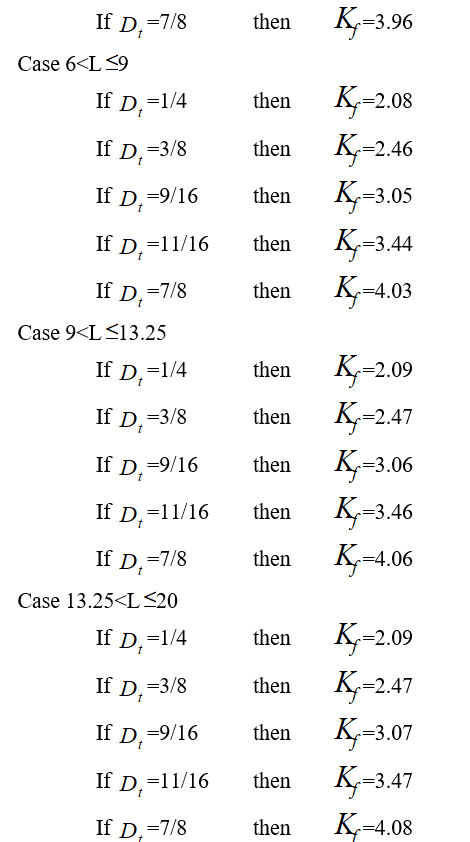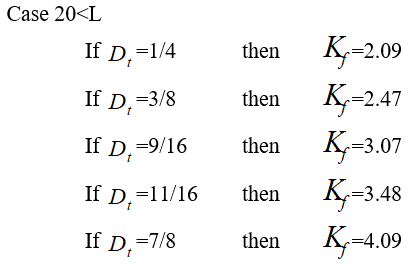5.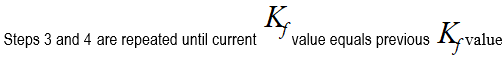6. Calculate natural frequency for L=L1: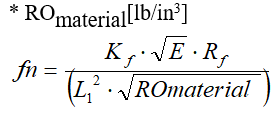7. Calculate wake frequency: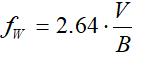8. Calculate magnification factor for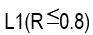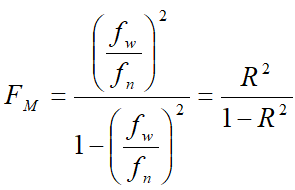9.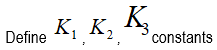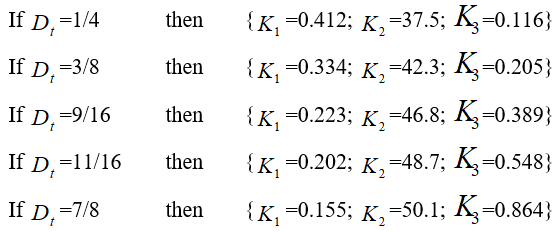10. Calculate Maximum Allowable Static Gage Pressure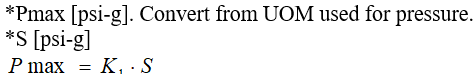11. Calculate maximum thermowell length based on steady state stress consideration.